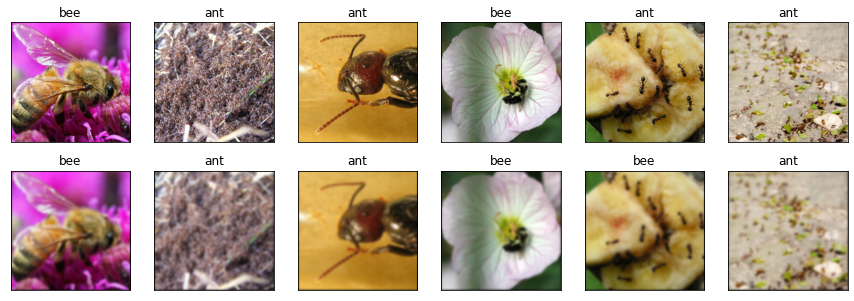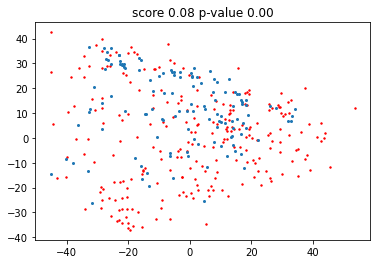# Drift detection on image classifiers¶

In this example, we take a simple classification model for image inputs and implement drift detection. As you can see below, the drift detection itself is very straightforward, perhaps even more so than the finetuning of the model.

In order to experiment, we need a dataset. We grab an ants versus bees dataset from the PyTorch tutorials.

Because we will want to apply transformations to simulate input drift, we split the PyTorch data loading chain a bit differently than usually done: Instead of normalizing the channels to zero mean / unit variance (on imagenet) in the dataset augmentation, we do this in an auxiliary function in the model just before feeding a batch.

We implement a LightningDataModule but with an important additional default_dataloader method that allows us to liberally choose the number of samples and the batch size. We will later use the additional transform to generate a drifted dataset on the fly.

:

torchvision.datasets.utils.download_and_extract_archive('https://download.pytorch.org/tutorial/hymenoptera_data.zip', 'data/')

# these are the standard transforms without the normalization (which we move into the model.step/predict before the forward)
train_transform = torchvision.transforms.Compose([
torchvision.transforms.RandomResizedCrop(size=(224, 224), scale=(0.08, 1.0), ratio=(0.75, 1.3333)),
torchvision.transforms.RandomHorizontalFlip(p=0.5),
torchvision.transforms.ToTensor()])
val_transform = torchvision.transforms.Compose([
torchvision.transforms.Resize(size=256),
torchvision.transforms.CenterCrop(size=(224, 224)),
torchvision.transforms.ToTensor()])

class OurDataModule(pl.LightningDataModule):
if parent is None:
self.train_dataset = torchvision.datasets.ImageFolder('./data/hymenoptera_data/train/',
transform=train_transform)
self.val_dataset = torchvision.datasets.ImageFolder('./data/hymenoptera_data/val/',
transform=val_transform)
self.test_dataset = torchvision.datasets.ImageFolder('./data/hymenoptera_data/test/',
transform=val_transform)
self.train_batch_size = 4
self.val_batch_size = 128
else:
self.train_dataset = parent.train_dataset
self.val_dataset = parent.val_dataset
self.test_dataset = parent.test_dataset
self.train_batch_size = parent.train_batch_size
self.val_batch_size = parent.val_batch_size

self.prepare_data()
self.setup('fit')
self.setup('test')

def setup(self, typ):
pass

def collate_fn(self, batch):
batch = torch.utils.data._utils.collate.default_collate(batch)
return batch

num_workers=4, shuffle=True, collate_fn=self.collate_fn)
num_workers=4, shuffle=False, collate_fn=self.collate_fn)
num_workers=4, shuffle=False, collate_fn=self.collate_fn)

dataset = self.val_dataset
if batch_size is None:
batch_size = self.val_batch_size
replacement = num_samples is not None
if shuffle:
sampler = torch.utils.data.RandomSampler(dataset, replacement=replacement, num_samples=num_samples)
else:
sampler = None
collate_fn=self.collate_fn)

datamodule = OurDataModule()


Using downloaded and verified file: data/hymenoptera_data.zip
Extracting data/hymenoptera_data.zip to data/


## Build a model¶

We use the TorchVision ResNet18 as our starting point.

Note that while we train a model here, in the end we use only the feature extractor for the drift detection, but we want to create the realistic situation where you have a classification model. Nothing here is specific to drift detection except.

:

feature_extractor = torchvision.models.resnet18(pretrained=True)
feature_extractor.fc = torch.nn.Identity()

:

class Classifier(pl.LightningModule):
def __init__(self, base_classifier):
super().__init__()
self.backbone = base_classifier
self.backbone.eval()
for p in self.backbone.parameters():
self.classifier = torch.nn.Linear(512, 2)

def normalize(self, x: torch.Tensor):
# We pull the normalization, usually done in the dataset into the model forward
x = torchvision.transforms.functional.normalize(x, mean=[0.485, 0.456, 0.406], std=[0.229, 0.224, 0.225])
return x

def forward(self, x: torch.Tensor):
x = self.normalize(x)
y = self.backbone(x)
return self.classifier(y)

def training_step(self, batch: torch.Tensor, batch_idx: int):
x, y = batch
y_hat = self(x)
loss = torch.nn.functional.cross_entropy(y_hat, y)
acc = (y_hat.max(1).indices == y).float().mean()
self.log('train_loss', loss)
self.log('train_acc', acc)
return loss

def validation_step(self, batch: torch.Tensor, batch_idx: int):
x, y = batch
y_hat = self(x)
loss = torch.nn.functional.cross_entropy(y_hat, y)
acc = (y_hat.max(1).indices == y).float().mean()
self.log('val_loss', loss)
self.log('val_acc', acc)
return loss

def test_step(self, batch: torch.Tensor, batch_idx: int):
x, y = batch
y_hat = self(x)
loss = torch.nn.functional.cross_entropy(y_hat, y)
acc = (y_hat.max(1).indices == y).float().mean()
self.log('test_loss', loss)
self.log('test_acc', acc)
return loss

def predict(self, batch: Any, batch_idx: Optional[int]=None, dataloader_idx: Optional[int] = None):
return self(batch)

def configure_optimizers(self):

model = Classifier(feature_extractor)


We finetune our network a bit (we set the requires grad to False for the resnet):

:

trainer = pl.Trainer(max_epochs=3, gpus=1, checkpoint_callback=False, logger=False)
trainer.fit(model, datamodule)

GPU available: True, used: True
TPU available: None, using: 0 TPU cores
LOCAL_RANK: 0 - CUDA_VISIBLE_DEVICES: 

| Name       | Type   | Params
--------------------------------------
0 | backbone   | ResNet | 11.2 M
1 | classifier | Linear | 1.0 K
--------------------------------------
1.0 K     Trainable params
11.2 M    Non-trainable params
11.2 M    Total params
44.710    Total estimated model params size (MB)
Widget Javascript not detected.  It may not be installed or enabled properly.

Widget Javascript not detected.  It may not be installed or enabled properly.

Widget Javascript not detected.  It may not be installed or enabled properly.

Widget Javascript not detected.  It may not be installed or enabled properly.

Widget Javascript not detected.  It may not be installed or enabled properly.

:

1


Let us check that things are OK. You should get 85%-95% accuracy.

:


trainer.test(model, datamodule=datamodule)

Widget Javascript not detected.  It may not be installed or enabled properly.

--------------------------------------------------------------------------------
{'test_acc': 0.9607843160629272, 'test_loss': 0.20751166343688965}
--------------------------------------------------------------------------------

:

[{'test_loss': 0.20751166343688965, 'test_acc': 0.9607843160629272}]


## Simulating drifted data¶

For systematic experiments, we want to compare the output of the drift detector on benign (non-drifted) and and drifted, here (partially) out of distribution samples. We simulate out of distribution data by applying a gaussian blur. In reality you might have effects like the camera lense losing focus or dirt impeding the picture quality.

Note that we do not use the drifted data for “training” the drift detector, but just for evaluation!

On the technical side, we take our datamodule as the in-distribution datamodule as is and use a derived datamodule which applies the gaussian blur in addition to the usual transforms as the out of distribution datamodule.

:

def corruption_function(x: torch.Tensor):

ind_datamodule = datamodule


Let us grab a few inputs and show them. We also let our model predict labels. Sometimes we see that labels are flipped for the blurred images.

:

inputs, _ = next(iter(datamodule.default_dataloader(shuffle=True)))
inputs_ood = corruption_function(inputs)

N = 6
model.eval()
inps = torch.cat([inputs[:N], inputs_ood[:N]])
model.cpu()
predictions = model.predict(inps).max(1).indices

predicted_labels = [["ant","bee"][p] for p in predictions]
pyplot.figure(figsize=(15, 5))
for i in range(2 * N):
pyplot.subplot(2, N, i + 1)
pyplot.title(predicted_labels[i])
pyplot.imshow(inps[i].permute(1, 2, 0))
pyplot.xticks([])
pyplot.yticks([])For drift detection, we need a feature extractor (of course, we had one above, too, but let’s play along and pretend we got the model from our colleague).

:

feature_extractor = copy.deepcopy(model)
feature_extractor.classifier = torch.nn.Identity()



## The drift detector¶

We instantiate the drift detector. In this example we use the Kernel MMD drift detector on the features extracted by the pretrained ResNet. We believe this is a good choice for your initial experiments, too.

:

drift_detector = torchdrift.detectors.KernelMMDDriftDetector()


We fit the drift detector using benign training data only. If you have a more sizeable dataset, you likely want to limit the number of samples you use here, you can do this by specifying the num_batches parameter to the fit function.

:

torchdrift.utils.fit(datamodule.train_dataloader(), feature_extractor, drift_detector)

100%|██████████| 61/61 [00:01<00:00, 36.39it/s]


We can then put the drift detector to action. TorchDrift uses vanilla torch.nn.Modules so you can just build a Sequential of the feature extractor and drift detector (or, in other use-cases an additional dimension reduction step in between).

:

drift_detection_model = torch.nn.Sequential(
feature_extractor,
drift_detector
)


First we test the output on benign input. By our setup (see the overview for details), we expect the computed p-value to be uniformely distributed on the unit interval $$[0, 1]$$, so with 95% probability, it is above 0.05. Note that for the p-value lower means “more likely that things have drifted”, while the same leads to higher scores.

:

features = feature_extractor(inputs)
score = drift_detector(features)
p_val = drift_detector.compute_p_value(features)
score, p_val

:

(tensor(0.0129), tensor(0.0520))


We can also visualize the two distribution to detemine whether we “intuitively believe” that the two samples are from the same distribution.

You may wonder why we use Isomap here instead of the more well-known (in certain circles) t-SNE. The reason is that Isomap actually gives us a transformation (from the training data) that we can use on the inputs.

:

N_base = drift_detector.base_outputs.size(0)
mapper = sklearn.manifold.Isomap(n_components=2)
base_embedded = mapper.fit_transform(drift_detector.base_outputs)
features_embedded = mapper.transform(features)
pyplot.scatter(base_embedded[:, 0], base_embedded[:, 1], s=2, c='r')
pyplot.scatter(features_embedded[:, 0], features_embedded[:, 1], s=4)
pyplot.title(f'score {score:.2f} p-value {p_val:.2f}');We can also do the same with out of distribution data. Typically the p-value here is very low - that it is actually 0 is an artifact from the bootstrapping we use for computing the p-value.

Can you spot a difference in the plot?

:

features = feature_extractor(inputs_ood)
score = drift_detector(features)
p_val = drift_detector.compute_p_value(features)

features_embedded = mapper.transform(features)
pyplot.scatter(base_embedded[:, 0], base_embedded[:, 1], s=2, c='r')
pyplot.scatter(features_embedded[:, 0], features_embedded[:, 1], s=4)
pyplot.title(f'score {score:.2f} p-value {p_val:.2f}');This concludes our overview.

Here, we saw how to get scores and p-values for the drift detection, taking the feature extractor from our classification model (our you might use an imagenet-trained feature extractor regardless of your model).

We show some more systematic experimentation in Comparing Drift Detectors. Also check our our example how to use the drift detector in monitoring a model.

View this document as a notebook: https://github.com/torchdrift/torchdrift/blob/master/notebooks/drift_detection_on_images.ipynb